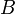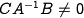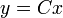Errata: In Exercise 6.4, need to assume A is invertible and C A^-1 B is nonzero

If the vectoris not in the range ofthen additional assumptions are required in order to show the desired result: Assume that the matrixis invertible and. In addition, it should be shown that the output error (with) goes to zero (as opposed to the state error).